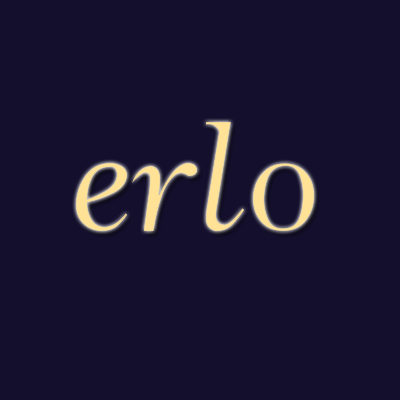### ×打赏# Python描述符的使用

## 场景介绍

``````
class Product():

def __init__(self,name,quantity,price):
self.name = name
self.quantity = quantity
self.price = price
``````

``````
class Product():

def __init__(self,name,quantity,price):
self.name = name
if quantity<0:
raise ValueError('quantity must be >= 0')
self.quantity = quantity
if quantity<0:
raise ValueError('price must be >= 0')
self.price = price
``````

``````
class Product():

def __init__(self,name,quantity,price):
self.name = name
self.quantity = quantity
self.price = price

@property
def quantity(self):
return self._quantity

@quantity.setter
def quantity(self,value):
if value < 0:
raise ValueError('quantity must be >= 0')
else:
self._quantity = value

@property
def price(self):
return self._price

@price.setter
def price(self, value):
if value < 0:
raise ValueError('price must be >= 0')
else:
self._price = value

book = Product('mybook',6,30)
print(book.quantity)
``````

## 使用描述符

(这几个方法是特殊方法，双下划线由于转换未显示)

``````
class NotNegative():
def __init__(self,name):
self.name = name

def __set__(self, instance, value):
if value < 0:
raise ValueError(self.name+' must be >= 0')
else:
instance.__dict__[self.name] = value

class Product():
quantity = NotNegative('quantity')
price = NotNegative('price')

def __init__(self,name,quantity,price):
self.name = name
self.quantity = quantity
self.price = price

book = Product('mybook',2,5)
``````

NotNegative是描述符类，它是Product类的类属性

• self ：是描述符实例

• instance ：是相当于例子中的实例book

• value ：就是要赋予的值

get方法同样有3个参数self, instance, owner。self，instance与set中的相同，owner为例子中的Product类

``````
instance.__dict__[self.name] = value
``````

### Erlo大厅()

* 这里是“吐槽厅”，所有人可看，只保留当天信息。

• ###Erlo.vip2019-05-22 16:09:07Hello、欢迎使用吐槽厅，这里是个吐槽的地方。
• 首页 笔记分享 案例展示 ERLO 搜索
鼠标试试
返回顶部